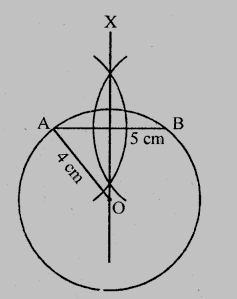# A line segment $AB$ is of length $5\ cm$. Draw a circle of radius $4\ cm$ passing through $A$ and $B$. Can you draw a circle of radius $2\ cm$ passing through $A$ and $B$? Give reason in support of your answer.

Given:

A line segment $AB$ is of length $5\ cm$.

To do:

We have to draw a circle of radius $4\ cm$ passing through $A$ and $B$ and if possible a circle of radius $2\ cm$ passing through $A$ and $B$.

Solution:Steps of construction:

1. Draw a line segment $AB = 5\ cm$.

2. Draw a perpendicular bisector of $AB$.

3. With centre $A$ and radius $4\ cm$, draw an arc which intersects the perpendicular bisector at $O$.

4. With centre $O$ and radius $4\ cm$, draw a circle which passes through $A$ and $B$.

With radius $2\ cm$, we cannot draw the circle passing through $A$ and $B$ as the diameter ($2 + 2 = 4\ cm$) is shorter than $5\ cm$.

Updated on: 10-Oct-2022

45 Views